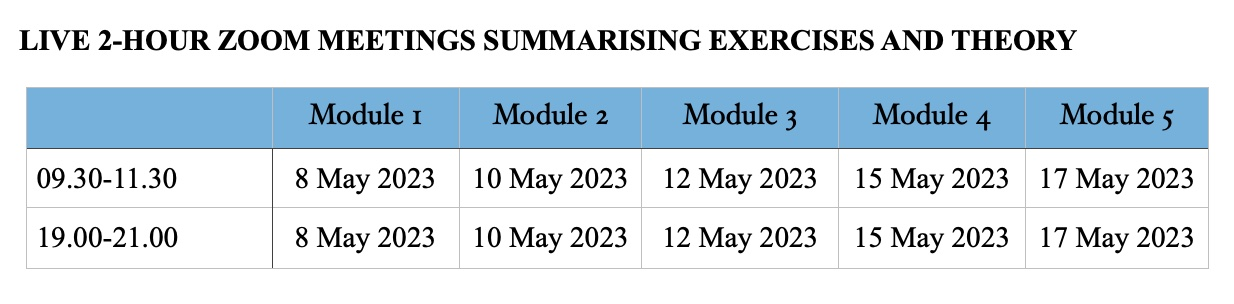# Online course 2: Data exploration, multiple linear regression, GLM, and GAM. With an introduction to R. With Live Zoom sessions in May 2023.

£ 500.00 each

Flyer for this course

See the online flyer for a detailed description.

Format of the course

This online course consists of 5 modules representing a total of approximately 40 hours of work. Each module consists of short theory presentations, followed by exercises using real data sets. On-demand video files for the theory presentations and the exercises are available. We will summarise each module in a 2-hour Zoom session. The dates and times for the five Zoom sessions of the May 2023 course are given in the table below.These are UK times. The content of the morning and evening sessions is the same. If you want, you can start this course at an earlier (or later) date.

General outline

We begin with an introduction to R and provide a protocol for data exploration to avoid common statistical problems. We will discuss how to detect outliers, deal with collinearity and transformations.

An important statistical tool is multiple linear regression. Various basic linear regression topics will be explained from a biological point of view. We will discuss potential problems and show how generalised linear models (GLM) can be used to analyse count data, presence-absence data and proportional data. Sometimes, parametric models (linear regression, GLM) do not quite fit the data and in such cases generalised additive models (GAM; a smoothing technique) can be used.

Detailed outline

Module 1 consists of 5 on-demand videos

• General introduction.
• Introduction to R.
• Theory presentation on data exploration.
• Two exercises on data exploration.

Module 2 consists of 4 on-demand videos

• Theory presentation bivariate linear regression.
• Exercise on bivariate linear regression.
• Theory presentation multiple linear regression.
• One exercise.

Module 3 consists of 7 on-demand video files

• Theory presentation one interactions in multiple linear regression models.
• One exercise.
• Theory presentation on Poisson and negative binomial distributions.
• Theory presentation on Poisson GLM.
• Exercise Poisson GLM.
• Theory presentation on negative binomial GLM.
• Exercise negative binomial GLM.

Module 4 consists of 3 on-demand video files

• Theory presentation Bernoulli and binomial GLMs.
• Exercise Bernoulli GLM.
• Exercise binomial GLM.
• Introduction to DHARMa.

Module 5 consists of 6 on-demand video files

• Theory presentation on GAM.
• Exercises using Gaussian GAM.
• Exercise using Poisson GAM.
• Exercise using negative binomial GAM.
• Exercise using Bernoulli GAM.
• What to present in a paper

Course material is based on:

• Zuur, Ieno and Smith (2007). Analysis Ecological Data. Springer.
• Zuur, Ieno, Elphick. (2010). A protocol for data exploration to avoiding common statistical problems. Methods in Ecology and Evolution, 1: 3-14.
• Zuur (2013) Beginner’s Guide to GAM with R.
• Zuur, Hilbe, Ieno (2013). Beginner’s Guide to GLM and GLMM with R.

Free 1-hour face-to-face video meeting

The course fee includes a 1-hour face-to-face video meeting with one or both instructors. The meeting needs to take place within 12 months after the last live zoom meeting. You can discuss your own data but we strongly suggest that the statistical topics are within the content of the course. The 1-hour needs to be used in one session and will take place on a mutually convenient day.

Pre-required knowledge

Basic statistics (e.g. mean, variance, normality). No R knowledge is required. You will learn R ‘on the fly’. This is a non-technical course.

Cancellation policy: See flyer